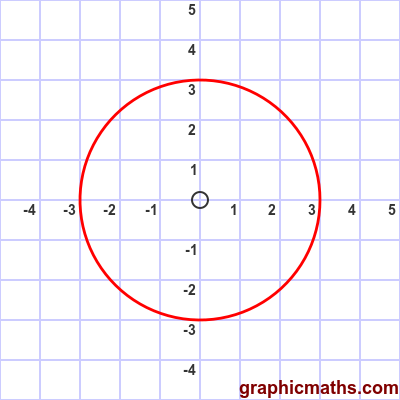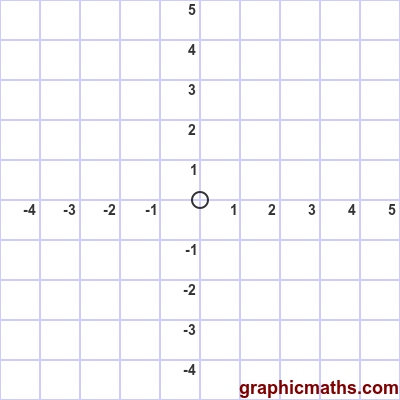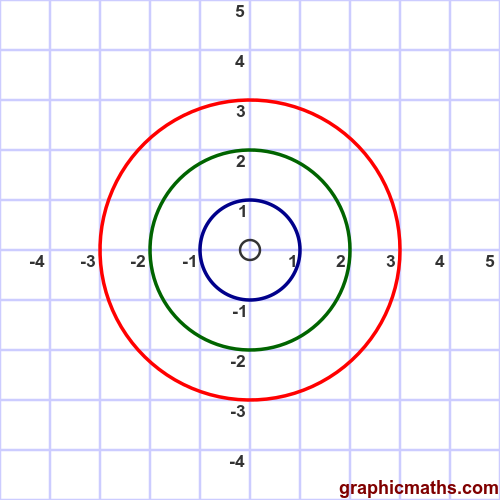# Polar curve constant r

By Martin McBride, 2020-09-18
Tags: standard curves
Categories: polar coordinatesHere is an example of a polar function of constant $r$.

$$r = 3$$

This function describes a curve where $r$ is constant for any angle $\theta$, in this case 3. The curve represents the locus all the points that are a distance of exactly 3 from the origin. This curve, of course, is a circle of radius 3:This animation shows how the curve is plotted as $\theta$ moves from 0 to $2\pi$:In general the function:

$$r = n$$

where $n$ is a non-negative constant, describes a circle of radius $n$. Here are the circles created for $n = 3$ (red), $n = 2$ (green), and $n = 1$ (blue):## Cartesian equation

We can convert the polar equation into a Cartesian equation using the identity:

$$r^2 = x^2 + y^2$$

Substituting for $r$ in the equation above gives:

$$x^2 + y^2 = n^2$$

which is the Cartesian equation for a circle of radius $n$, centred on the origin.